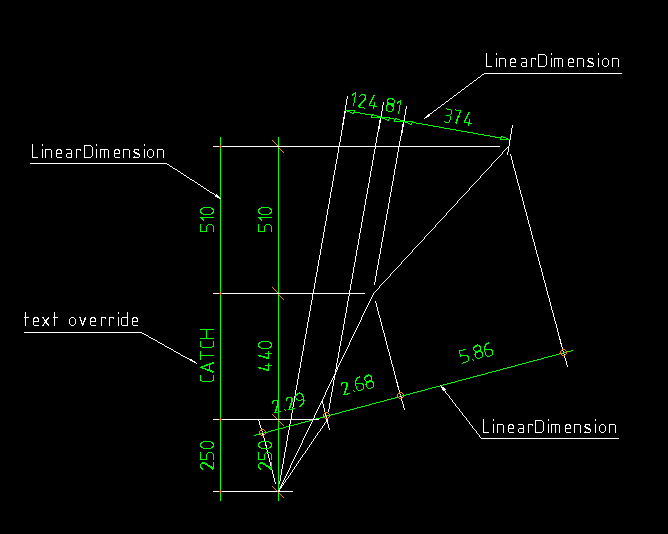# LinearDimension¶

Type: Composite Entity

class `LinearDimension`

Simple straight dimension line with two or more measure points, build with basic DXF entities. This is NOT a dxf dimension entity. And This is a 2D element, so all z-values will be ignored!

`LinearDimension.``__init__`(pos, measure_points, angle=0., dimstyle='Default', layer=None, roundval=None)
Parameters: pos – location as (x, y) tuple of dimension line, line goes through this point measure_points – list of points as (x, y) tuples to dimension (two or more) angle (float) – angle (in degree) of dimension line dimstyle (str) – dimstyle name, ‘Default’ - style is the default value layer (str) – dimension line layer, override the default value of dimstyle roundval (int) – count of decimal places

## Methods¶

`LinearDimension.``set_text`(section, text)

Set and override the text of the dimension text for the given dimension line section.

## Properties¶

`LinearDimension.``section_count`

count of dimline sections

`LinearDimension.``point_count`

count of dimline points

## Example¶

```import dxfwrite
from dxfwrite import DXFEngine as dxf

# Dimlines are separated from the core library.
# Dimension lines will not generated by the DXFEngine.
from dxfwrite.dimlines import dimstyles, LinearDimension

# create a new drawing
dwg = dxf.drawing('dimlines.dxf')

# dimensionline setup:
# add block and layer definition to drawing
dimstyles.setup(dwg)

# create a dimension line for following points
points = [ (1.7,2.5), (0,0), (3.3,6.9), (8,12)]

# define new dimstyles, for predefined ticks see dimlines.py
dimstyles.new("dots", tick="DIMTICK_DOT", scale=1., roundval=2, textabove=.5)
dimstyles.new("arrow", tick="DIMTICK_ARROW", tick2x=True, dimlineext=0.)
dimstyles.new('dots2', tick="DIMTICK_DOT", tickfactor=.5)